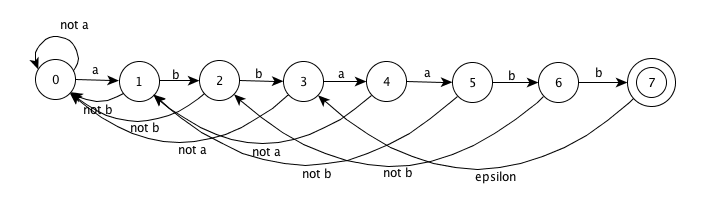• Introduction
• 1. 1.Introduction
• 2. 2.A Simple Syntax-Directed Translator
• 3. 3.Lexical Analysis
• 4. 4.Syntax Analysis
• 5. 5.Syntax-Directed Translation
• 6. 6.Intermediate-Code Generation
• 7. 7.Run-Time Environments
• 8. 8.Code Generation
• 9. 12.InterProcedural Analysis
• Published with GitBook

# 3.6 Exercises for Section 3.6

### 3.6.1 !

Figure 3.19 in the exercises of Section 3.4 computes the failure function for the KMP algorithm. Show how, given that failure function, we can construct, from a keyword b1b2...bn an n + 1-state DFA that recognizes .*b1b2...bn, where the dot stands for "any character." Moreover, this DFA can be constructed in O(n) time.

Take the string "abbaabb" in exercise 3.4.3-3 as example, the failure function is:

• n : 1, 2, 3, 4, 5, 6, 7
• f(n): 0, 0, 0, 1, 1, 2, 3

The DFA is：Pseudocode of building the DFA：

``````for (i = 0; i< n; i ++) {
move[s[i], c] = {
if ( c == b1b2…bn[i] ) {
goto s[i+1]
} else {
goto s[f(i)]
}
}
}
``````

It is obviously that with the known f(n), this DFA can be constructed in O(n) time.

### 3.6.2

Design finite automata (deterministic or nondeterministic) for each of the languages of Exercise 3.3.5.

### 3.6.3

For the NFA of Fig. 3.29, indicate all the paths labeled aabb. Does the NFA accept aabb?

• (0) -a-> (1) -a-> (2) -b-> (2) -b-> ((3))
• (0) -a-> (0) -a-> (0) -b-> (0) -b-> (0)
• (0) -a-> (0) -a-> (1) -b-> (1) -b-> (1)
• (0) -a-> (1) -a-> (1) -b-> (1) -b-> (1)
• (0) -a-> (1) -a-> (2) -b-> (2) -b-> (2)
• (0) -a-> (1) -a-> (2) -b-> (2) -ε-> (0) -b-> (0)
• (0) -a-> (1) -a-> (2) -ε-> (0) -b-> (0) -b-> (0)

This NFA accepts "aabb"

### 3.6.4

Repeat Exercise 3.6.3 for the NFA of Fig. 3.30.

### 3.6.5

Give the transition tables for the NFA of:

1. Exercise 3.6.3.
2. Exercise 3.6.4.
3. Figure 3.26.

Table 1

state a b ε
0 {0,1} {0}
1 {1,2} {1}
2 {2} {2,3} {0}
3

Table 2

state a b ε
0 {1} {3}
1 {2} {0}
2 {3} {1}
3 {0} {2}

Table 3

state a b ε
0 {1,2}
1 {2}
2 {2}
3 {4}
4 {4}